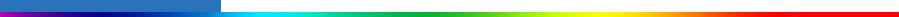Working Group I: The Scientific BasisGet Javascript Other reports in this collection

#### 6.8.2.2 Estimates of the second indirect effect and of the combined effect

Whereas the first indirect effect can be computed diagnostically, assessment of the second indirect effect implies that two independent (i.e., with the same fixed sea surface temperatures, but with different meteorologies) GCM simulations be made, a first one with pre-industrial and a second one with present day aerosols. The difference in top of the atmosphere fluxes or cloud radiative forcings between two such simulations is used as a proxy for the forcings due to the second indirect and the combined effects. The simulations need to be sufficiently long (usually 5 years) so that the effects of natural variability are expected to average out. However, as a consequence, the estimates of the aerosol indirect effect may include some undesirable feedbacks involving climate parameters which would violate the definition adopted for radiative forcing of climate change by IPCC (see also Section 6.1.1). Rotstayn and Penner (2001) showed that, at least in their model, the difference in top of the atmosphere fluxes between two simulations did not differ by more than 10% from the radiative forcing for the first indirect effect computed diagnostically. Caution should nevertheless be exercised before this result can be generalised to other models and to the second indirect effect.

Jones et al. (1999) and Rotstayn (1999) provide estimates for the second indirect effect alone with ranges of -0.53 to -2.29 Wm-2 and -0.4 to -1.0 Wm-2, respectively. For the combined effect (first and second effects estimated together), Jones et al. (1999) and Rotstayn (1999) give best estimates of -1.18 and -2.1 Wm-2. Larger radiative impacts are found in sensitivity tests by Rotstayn (1999) and Lohmann and Feichter (1997), with values of -3.2 and -4.8 Wm-2, respectively. Rotstayn (1999) tried an alternative parametrization for cloud droplet concentration (Roelofs et al., 1998) and Lohmann and Feichter (1997) tried an alternative cloud scheme. In contrast to these studies, Lohmann et al. (2000) adopted a “mechanistic” approach, where they introduced a prognostic equation for the cloud droplet number concentration. They predict a much smaller combined effect with radiative impact of 0.0 and -0.4 Wm-2, assuming externally and internally mixed sulphate aerosols, respectively. The authors attribute this rather small radiative impact to the small increase in anthropogenic sulphate aerosol number concentrations. Ghan et al. (2001a) estimate a combined effect of –1.7 Wm-2 using a mechanistic approach similar to that of Lohmann et al., but with aerosol size distribution predicted rather than prescribed. The larger forcing is due to the absence of lower bounds on droplet and aerosol number concentrations in the simulations by Ghan et al. (2001a). Studies by Rotstayn (1999) and Jones et al. (1999) both indicate that the positive long-wave forcing associated with the indirect aerosol effect is small (between 0.0 and 0.1 Wm-2 for each of the effects).

The partitioning of the total indirect radiative impact between the first and second effect is uncertain. Jones et al. (1999) found that the ratio between their best estimates of the first and second indirect effects, taken separately, was 1.38, while Lohmann et al. (2000) predicted a ratio of 0.71 (for sulphate and carbonaceous aerosols taken together). Rotstayn (1999) and Lohmann et al. (2000) estimated that the radiative impact for the combined effects was of similar magnitude than the sum of the two effects considered separately. In contrast, Jones et al. (1999) found that the combined radiative impact (-1.18 Wm-2) was less than the sum of the two effects (estimated as -0.91 and -0.66 Wm-2, respectively, yielding a sum of -1.57 Wm-2).

#### 6.8.2.3 Further discussion of uncertainties

Some authors have argued that sea salt particles may compete with sulphate aerosols as cloud condensation nuclei, thereby reducing the importance of anthropogenic sulphate in droplet nucleation (Ghan et al., 1998; O’Dowd et al., 1999). While this process is empirically accounted for in some of the above mentioned estimates (e.g., Jones et al., 1999), it certainly adds further uncertainty to the estimates. Considerable sensitivity is found to the parametrization of the autoconversion process (Boucher et al., 1995; Lohmann and Feichter, 1997; Delobbe and Gallée, 1998; Jones et al., 1999) which complicates matters because there is a need to “tune” the autoconversion onset in GCMs (Boucher et al., 1995; Fowler et al., 1996; Rotstayn, 1999, 2000) to which the indirect aerosol forcing is sensitive (Jones et al., 1999; Rotstayn, 1999; Ghan et al., 2001a). The indirect aerosol forcing is also sensitive to the treatment of the pre-industrial aerosol concentration and properties (Jones et al., 1999; Lohmann et al., 2000) which remain poorly characterised, the representation of the microphysics of mid-level clouds (Lohmann et al., 2000), the representation of aerosol size distribution (Ghan et al., 2001a), the parametrization of sub-grid scale clouds, the horizontal resolution of the GCM (Ghan et al., 2001a), and the ability of GCMs to simulate the stratocumulus cloud fields. Finally, it should be noted that all the studies discussed above cannot be considered as truly “independent” because many of them (with the exceptions of Lohmann et al. (2000) and Ghan et al. (2001a)) use similar methodologies and similar relationships between sulphate mass and cloud droplet number concentration. Therefore it is suspected that the range of model results does not encompass the total range of uncertainties. In an overall sense, it can be concluded that the considerable sensitivities to the treatment of microphysical details associated with aerosol-cloud interactions, and their linkages with macroscopic cloud and circulation parameters, remain to be thoroughly explored.